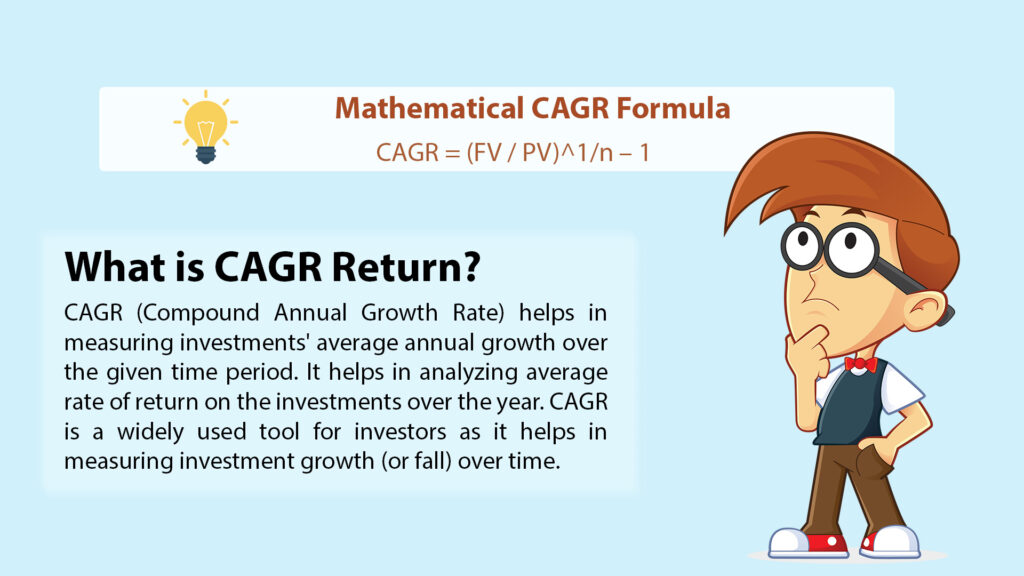# What is CAGR Return?CAGR (Compound Annual Growth Rate) helps in measuring investments’ average annual growth over the given time period. It helps in analyzing average rate of return on the investments over the year. CAGR is a widely used tool for investors as it helps in measuring investment growth (or fall) over time. When industry experts calculate CAGR, it is assumed that profits are reinvested at the end of each year of the time horizon. Thus, CAGR is a representative number, and is not an accurate return. In some of the cases, investment cannot grow at the similar rate year-over-year. Still, the CAGR calculator is being used to compare the alternative investments.

To calculate CAGR on investment, experts use the following steps:

1. Divide the investment value at the end by the initial value.
2. Increase the result to the power of 1 divided by ‘n’, which is tenure of investment in years.
3. Then, deduct one from the total value

Mathematically, the CAGR formula is calculated with the help of following equation-
CAGR = (FV / PV)^1/n – 1

CAGR Versus Absolute Returns
People look at their returns in absolute terms. These returns help the investor in knowing how much his investment has earned over this entire duration. Let us take one example. One investor has invested INR50,000 in a mutual fund in 2017. The value of the investment has increased to ₹85,000 in 2022.

In absolute terms, this investor earned a 70% return on the investment of INR50,000 over a 5-year period. Now, this sounds quite brilliant. However, it doesn’t give the full picture. The investor has no idea about the average rate of return earned on the investment every year. Therefore, it is utmost important to know how well the investments have performed on an average on a yearly basis. CAGR can prove to be very helpful in situations like these.